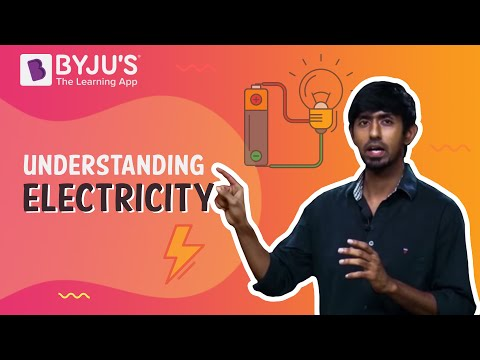# Class 10 Physics Chapter 12 Electricity MCQs

Class 10 Physics Chapter 12 Electricity MCQs are provided here with answers. Electricity is the fundamental concept of science. These MCQ questions are designed as per the latest CBSE syllabus and NCERT curriculum. Solving these chapter-wise MCQs will help students to score good marks in the final exam. Electricity Class 10 Science MCQs are prepared for a better understanding of the concept. It allows students to test their knowledge and answering skills in the given time frame.

## MCQs on Class 10 Physics Chapter 12 Electricity

Check the multiple-choice questions for the Class 10 Physics Chapter 12 Electricity. Each MCQ will have four options here, out of which only one is correct. Students have to pick the correct option and check the answer provided here.

Download Chapter 12 Electricity MCQs PDF by clicking on the button below.

1. Which of the following is the good conductor of electricity?

1. Paper
2. Iron
3. Glass
4. Ebonite

Explanation: Iron is a good conductor of electricity. Paper, Glass, and Ebonite are insulators which do not allow electricity to pass through them.

2. The SI unit of electric current is ———.

1. Volt
3. Ampere
4. Ohms

Explanation: The SI unit of electric current is Ampere.

3. Electrical resistivity of a metallic wire depends upon its ———.

1. Length
2. Material
3. Thickness
4. Shape

Explanation: Electrical resistivity of a metallic wire depends upon its thickness.

4. Electric current is measured using ————–.

1. Galvanometer
2. Ammeter
3. Voltmeter
4. Potentiometer

Explanation: Ammeter is a device used to measure electricity.

5. Unit of electric power may also be expressed as ————–.

1. Watt second
2. Ampere hour
3. Volt second
4. Kilowatt hour

Explanation: Unit of electric power is also expressed in Kilowatt hour.

6. Materials which do not allow electricity to pass through are known as —————–.

1. Semiconductor
2. Conductor
3. Insulator
4. Semi-insulator

Explanation: Insulators do not pass electric current through them.

7. Tungsten is used in which of the following?

1. Metal extraction
2. Electric bulb
3. Insulators
4. Textile manufacturing

Explanation: Tungsten filament is the main element in the electric bulb.

8. Electric potential is a ————.

1. Vector quantity
2. Scalar quantity
3. Dimensionless quantity
4. None of the option

Explanation: Since electric potential has only magnitude and no direction, it is a scalar quantity.

9. Copper is used in electric transmission lines because ———————-.

1. High resistivity
2. Low resistivity
3. No resistivity
4. None of the option

Explanation: Since copper offers low resistivity, it is an ideal material to be used in electric transmission.

10. The SI unit of resistance is ————.

1. Volt